Win up to 100% scholarship on Aakash BYJU'S JEE/NEET courses with ABNAT Win up to 100% scholarship on Aakash BYJU'S JEE/NEET courses with ABNAT

# JEE Main 2022 June 24 – Shift 2 Chemistry Question Paper with Solutions

The JEE Main 2022 June 24 Shift 2 Chemistry question paper with solutions is provided here. Students can go through the solutions given below for the JEE main 2022 chemistry June 24 – second shift question paper to verify the answers they wrote during the examination. The solutions for all the JEE main 2022 question papers are provided by our subject experts in an easily understandable way. Try solving the JEE main 2022 chemistry question papers, and verify your answers to excel in the upcoming exams. Get complete solutions and a printable PDF for the same on this page.
JEE Main 2022 June 26 – Shift 2 Chemistry Question Paper with Solutions

SECTION – A

Multiple Choice Questions: This section contains 20 multiple choice questions. Each question has 4 choices (1), (2), (3) and (4), out of which ONLY ONE is correct.

1. 120 g of an organic compound that contains only carbon and hydrogen gives 330 g of CO2 and 270 g of water on complete combustion. The percentage of carbon and hydrogen, respectively are

(A) 25 and 75

(B) 40 and 60

(C) 60 and 40

(D) 75 and 25

Sol. Mass of organic compound = 120 g

Mass of CO2 = 330 g

Moles of CO2 = 330/44= 7.5

Mass of carbon = 7.5 × 12 = 90 gm

Percentage of C

$$\begin{array}{l}=\frac{90\times100}{120}=75%\end{array}$$

Mass of H2O = 270 g

Moles of H2O = 270/18= 15

Mass of hydrogen = 15 × 2 = 30 gm

Percentage of H

$$\begin{array}{l}=\frac{30\times100}{120}=25%\end{array}$$

2. The energy of one mole of photons of radiation of wavelength 300 nm is (Given h = 6.63 × 10–34Js, NA = 6.02 × 1023 mol–1, c = 3 × 108 ms–1)

(A) 235 kJ mol–1

(B) 325 kJ mol–1

(C) 399 kJ mol–1

(D) 435 kJ mol–1

Sol. Wavelength of radiation = 300 nm

Photon energy

$$\begin{array}{l}=\frac{hc}{\lambda}\end{array}$$
$$\begin{array}{l}=\frac{6.63\times 10^{-34}\times 3 \times 10^8}{300 \times 10^{-19}}\end{array}$$

= 6.63 × 10–19 J

Energy of 1 mole of photons

= 6.63 × 10–19 × 6.02 × 1023 × 10–3

= 399 kJ

3. The correct order of bond orders of C22-, N22-, O22- is, respectively

(A) C22-< N22-< O22-

(B) O22-< N22-< C22-

(C) C22-< O22-< N22-

(D) N22-< C22-< O22-

Sol.

$$\begin{array}{l}C_2^{2-}:\sigma_{1s}^{2}~ \sigma_{1s}^{*2}~\sigma_{2s}^{2}~\sigma_{2s}^{*2}~\pi_{2p_x}^{2} = \pi_{2p_y}^{2}\sigma_{2p_z}^{2} \end{array}$$

$$\begin{array}{l}N_2^{2-}:\sigma_{1s}^{2}~ \sigma_{1s}^{*2}~\sigma_{2s}^{2}~\sigma_{2s}^{*2}~\sigma_{2p_z}^{2}\pi_{2p_x}^{2} = \pi_{2p_y}^{2}\pi_{2p_x}^{*1}=\pi_{2p_y}^{*1}\end{array}$$
$$\begin{array}{l}O_2^{2-}:\sigma_{1s}^{2}~ \sigma_{1s}^{*2}~\sigma_{2s}^{2}~\sigma_{2s}^{*2}~\sigma_{2p_z}^{2}\pi_{2p_x}^{2} = \pi_{2p_y}^{2}\pi_{2p_x}^{*2}=\pi_{2p_y}^{*2}\end{array}$$
$$\begin{array}{l}\text{B.O.}\left(C_2^{2-}\right)=3;~~\text{B.O.}\left(N_2^{2-}\right)=2;~~\text{B.O.}\left(O_2^{2-}\right)=1 \end{array}$$

4. At 25°C and 1 atm pressure, the enthalpies of combustion are as given below :

Substance

H2

C(graphite)

C2H6(g)

$$\begin{array}{l}\frac{D_c H^\circleddash}{kJmol^{-1}}\end{array}$$

–286.0

–394.0

–1560.0

The enthalpy of formation of ethane is

(A) +54.0 kJ mol–1

(B) –68.0 kJ mol–1

(C) –86.0 kJ mol–1

(D) +97.0 kJ mol–1

Sol.

$$\begin{array}{l}2C (graphite)+3H_2 (g)\to C_2H_6 (g)\end{array}$$
$$\begin{array}{l}\Delta H_r=+1560+2(-394)+3(-286)\end{array}$$
= –86.0 kJ mol–1

Enthalpy of formation of C2H6(g) = –86.0 kJ mol–1

5. For a first order reaction, the time required for completion of 90% reaction is ‘x’ times the half life of the reaction. The value of ‘x’ is (Given: In 10 = 2.303 and log 2 = 0.3010)

(A) 1.12

(B) 2.43

(C) 3.32

(D) 33.31

Sol.

$$\begin{array}{l}A\to Products\end{array}$$

For a first order reaction,

$$\begin{array}{l}t_{1/2}=\frac{ln 2 }{k}=\frac{0.693}{k}\end{array}$$

Time for 90% conversion,

$$\begin{array}{l}t_{90\%}=\frac{1}{k}In\frac{100}{10}=\frac{ln 10}{k}=\frac{2.303}{k}\end{array}$$
$$\begin{array}{l}t_{90\%}=\frac{2.303}{0.693}t_{1/2}=3.32t_{1/2}\end{array}$$

6. Metals generally melt at very high temperature. Amongst the following, the metal with the highest melting point will be

(1) Hg

(2) Ag

(3) Ga

(4) Cs

Sol. Melting points of the given metals

Hg : –38.83° C

Ag : 961.8° C

Ga : 29.76° C

Cs : 28.44° C

∴ Metal having highest melting point is Ag.

7. Which of the following chemical reactions represents Hall-Heroult Process?

(1) Cr2O3 + 2Al → Al2O3 + 2Cr

(2) 2Al2O3 + 3C → 4Al + 3CO2

(3) FeO + CO → Fe + CO2

(4)

$$\begin{array}{l}[2Au(CN)_2]_{aq}^{-}+Zn(s)\to 2Au(s)+[Zn(CN_4)]^{2-}\end{array}$$

Sol. Hall-Herault process is used for the extraction of aluminium by electrolysis molten Al2O3

2Al2O3 + 3C → 4Al + 3CO2

8. In the industrial production of which of the following, molecular hydrogen is obtained as a byproduct?

(1) NaOH

(2) NaCl

(3) Na metal

(4) Na2CO3

Sol. Molecular hydrogen is produced as a byproduct in the industrial production of NaOH by electrolysis of aq NaCl solution

NaCl → Na+ + Cl

$$\begin{array}{l}H_2O\rightleftharpoons H_+ + OH^-\end{array}$$

Cathode : 2H2O + 2e → H2 + 2OH

Anode : 2Cl → Cl2 +2e

NaOH is crystallised from the remaining part of electrolyte.

9. Which one of the following compounds is used as a chemical in certain type of fire extinguishers?

(1) Baking soda

(2) Soda ash

(3) Washing soda

(4) Caustic Soda

Sol. Baking soda (NaHCO3) is used in certain type of fire extinguishers because it decomposes at high temperature to produce CO2 which extinguishes fire

$$\begin{array}{l}2NaHCO_3(S)\overset{\Delta }{\rightarrow}Na_2CO_3(S)+H_2O\uparrow+CO_2\uparrow \end{array}$$

10. PCl5 is well known, but NCl5 is not. Because,

(1) nitrogen is less reactive than phosphorous

(2) nitrogen doesn’t have d-orbitals in its valence shell.

(3) catenation tendency is weaker in nitrogen than phosphorous.

(4) size of phosphorous is larger than nitrogen

Sol. PCl5 is well known but NCl5 is not because nitrogen does not have vacant d-orbitals in its valence shell. So, nitrogen cannot expand its octet. On the other hand phosphorus has vacant d-orbitals in its valence shell which enables it to expand its octet.

11. Transition metal complex with highest value of crystal field splitting (Δ0) will be

(A) [Cr(H2O)6]3+

(B) [Mo(H2O)6]3+

(C) [Fe(H2O)6]3+

(D) [Os(H2O)6]3+

Sol. Crystal field splitting (Δ0) for octahedral complexes depends on oxidation state of the metal as well as to which transition series the metal belongs. For the same oxidation state, the crystal field splitting (Δ0) increases as we move from 3d → 4d → 5d. Cr3+ and Fe3+ belong to 3d series, Mo3+ belongs to 4d series and Os3+ belongs to 5d series. Therefore crystal field splitting (Δ0) is highest for [Os(H2O)6]3+.

12. Some gases are responsible for heating of atmosphere (green house effect). Identify from the following the gaseous species which does not cause it.

(A) CH4

(B) O3

(C) H2O

(D) N2

Sol. Among the given gases, the green house gases which are responsible for heating the atmosphere are CH4, water vapour and ozone. Nitrogen is not a green house gas.

13. Arrange the following carbocations in decreasing order of stability.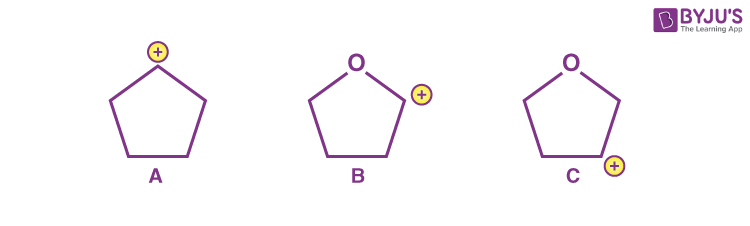(A) A > C > B

(B) A > B > C

(C) C > B > A

(D) C > A > B

Sol. The given carbocations areCarbocation (A) is stabilised by hyperconjugation due to 4 α hydrogen atoms. Carbocation (C) is also stabilised by hyperconjugation due to 4 α hydrogen atoms but destabilised by –I effect of O-atom. Carbocation (B) is most stable as it is stabilised by resonance.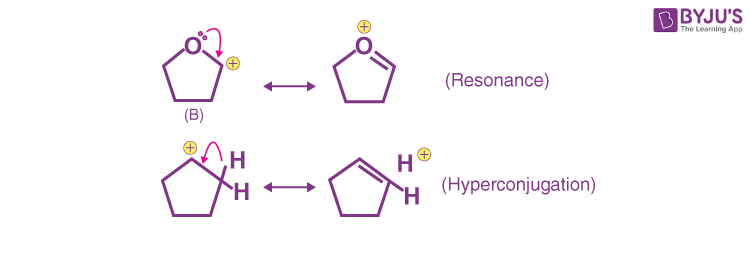∴ Correct decreasing order of stability is

B > A > C

None of the given options is correct.

14. Given below are two statements.

Statement I: The presence of weaker π-bonds make alkenes less stable than alkanes.

Statement II: The strength of the double bond is greater than that of carbon-carbon single bond.

In the light of the above statements, choose the correct answer from the options given below.

(A) Both Statement I and Statement II are correct.

(B) Both Statement I and Statement II are incorrect.

(C) Statement I is correct but Statement II is incorrect.

(D) Statement I is incorrect but Statement II is correct.

Sol. The π-bond present is alkenes is weaker than σ-bond present in alkanes. That makes alkenes less stable than alkanes. Therefore, statement-I is correct.

Carbon-carbon double bond is stronger than Carbon-carbon single bond because more energy is required to break 1 sigma and 1 pi bond than to break 1 sigma bond only. Therefore, statement-II is also correct.

15. Which of the following reagents/reactions will convert ‘A’ to ‘B’?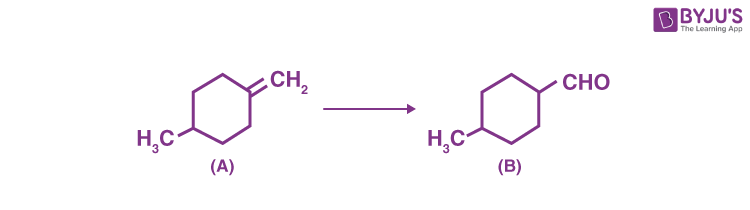(A) PCC oxidation

(B) Ozonolysis

(C) BH3, H2O2/OH followed by PCC oxidation

(D) HBr, hydrolysis followed by oxidation by K2Cr2O7.

Sol.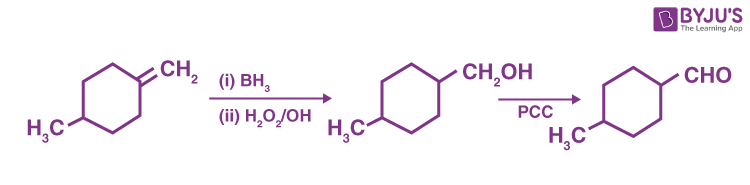The first step involves addition of H2O to alkene according to anti-markownikoff’s rule while the second step involves oxidation of 1° alcohol to aldehyde.

16. Hex-4-ene-2-ol on treatment with PCC gives ‘A’ on reaction with sodium hypoiodite gives ‘B’, which on further heating with soda lime gives ‘C’. The compound ‘C’ is

(A) 2-pentene

(B) Proponaldehyde

(C) 2-butene

(D) 4-methylpent-2-ene

Sol.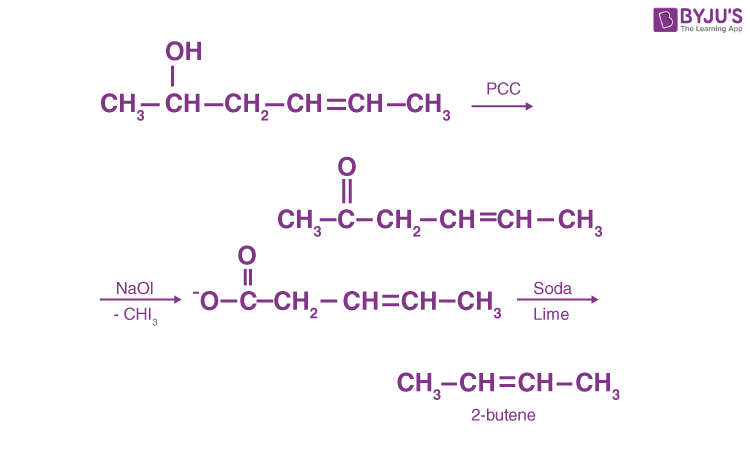17. The conversion of propan-1-ol to n-butylamine involves the sequential addition of reagents. The correct sequential order of reagents is

(A) (i) SOCl2 (ii) KCN (iii) H2/Ni, Na(Hg)/C2H5OH

(B) (i) HCl (ii) H2/Ni, Na(Hg)/C2H5OH

(C) (i) SOCl2 (ii) KCN (iii) CH3NH2

(D) (i) HCl (ii) CH3NH2

Sol.

$$\begin{array}{l}CH_3-CH_2-\underset{Propan-1-ol}{CH_2-OH\xrightarrow[]{SOCI_2}\end{array}$$
$$\begin{array}{l}CH_3-CH_2-CH_2-Cl\xrightarrow[]{KCN} \end{array}$$
$$\begin{array}{l}CH_3-CH_2-CH_2-CN\xrightarrow[Na(Hg)/C_2H_5OH]{H_2/Ni} \end{array}$$
$$\begin{array}{l}CH_3-CH_2-\underset{n-butylamine}{CH_2}-CH_2-NH_2 \end{array}$$

18. Which of the following is not an example of a condensation polymer?

(A) Nylon 6,6

(B) Dacron

(C) Buna-N

(D) Silicone

Sol. Nylon 6, 6 is a condensation polymer of hexamethylene diamine and adipic acid

Dacron is a condensation polymer of terephthalic acid and ethylene glycol.

Silicone is a condensation polymer of dialkyl silanediol.

19. The structure shown below is of which well-known drug molecule?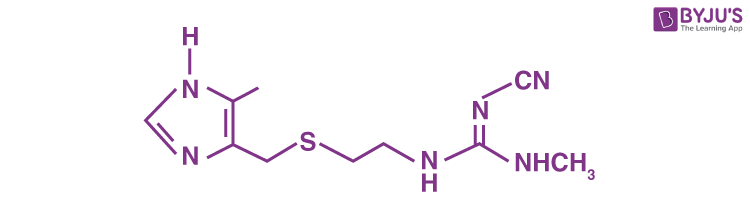(A) Ranitidine

(B) Seldane

(C) Cimetidine

(D) Codeine

Sol. The given structure is that of cimetidine which is well known antacid.

20. In the flame test of a mixture of salts, a green flame with blue centre was observed. Which one of the following cations may be present?

(A) Cu2+

(B) Sr2+

(C) Ba2+

(D) Ca2+

Sol. Cupric salts give green flame with blue centre. The colour of other salts are

Sr2+ Crimson red

Ca2+ Brick red

Ba2+ Green

SECTION – B

Numerical Value Type Questions: This section contains 10 questions. In Section B, attempt any five questions out of 10. The answer to each question is a NUMERICAL VALUE. For each question, enter the correct numerical value (in decimal notation, truncated/rounded-off to the second decimal place; e.g. 06.25, 07.00, –00.33, –00.30, 30.27, –27.30) using the mouse and the on-screen virtual numeric keypad in the place designated to enter the answer.

1. At 300 K, a sample of 3.0 g of gas A occupies the same volume as 0.2 g of hydrogen at 200 K at the same pressure. The molar mass of gas A is ______ g mol–1. (nearest integer) Assume that the behaviour of gases as ideal.

(Given: The molar mass of hydrogen (H2) gas is
2.0 g mol–1).

Sol. V1, Volume of 0.2 g H2 at 200 K

$$\begin{array}{l}=\frac{0.2\times R\times200}{2\times P} \end{array}$$

V2, Volume of 3.0 g of gas A at 300 K

$$\begin{array}{l}=\frac{0.3\times R\times300}{M\times P} \end{array}$$

V1 = V2 (Given)

$$\begin{array}{l}\frac{0.2\times R\times200}{2\times P}=\frac{3.0\times R\times300}{M\times P} \end{array}$$

∴ M = 45 g mol–1

2. A company dissolves ‘x’ amount of CO2 at 298 K in 1 litre of water to prepare soda water. X = _____
× 10–3 g. (nearest integer)

(Given: partial pressure of CO2 at 298 K = 0.835 bar.

Henry’s law constant for CO2 at 298 K = 1.67 kbar.

Atomic mass of H, C and O is 1, 12, and 6 g mol–1, respectively)

Sol. According to Henry’s law, partial pressure of a gas is given by

Pg = (KH) Xg

where Xg is mole fraction of gas in solution

$$\begin{array}{l}0.835 = 1.67\times 10^3\left ( X_{CO_{2}}\right)\end{array}$$
$$\begin{array}{l}\left ( X_{CO_2}\right)=5\times10^{-4} \end{array}$$

Mass of CO2 in 1 L water = 1221 × 10–3 g

3. PCl5 dissociates as

$$\begin{array}{l}PCl_5(g) \rightleftharpoons PCl_3(g) + Cl_2(g)\end{array}$$

5 moles of PCl5 are placed in a 200 litre vessel which contains 2 moles of N2 and is maintained at 600 K. The equilibrium pressure is 2.46 atm. The equilibrium constant Kp for the dissociation of PCl5 is ______ × 10–3. (nearest integer)

(Given: R = 0.082 L atm K–1 mol–1; Assume ideal gas behaviour)

Sol.

$$\begin{array}{l}PCl_5(g) \rightleftharpoons PCl_3(g) + Cl_2(g)\end{array}$$

Initial 5

moles

Equilibrium 5 – x x x

moles

Number of moles of N2 = 2

Equilibrium pressure = 2.46 atm

$$\begin{array}{l} P_{eq} = \frac{(7+x)\times0.082\times600)}{200}=2.46\end{array}$$

On solving, x = 3

$$\begin{array}{l}\therefore K_p = \frac{\left (\frac{3P}{10}\right)\left(\frac{3P}{10}\right)}{\frac{2P}{10}}=\frac{9\times2.46}{20}\end{array}$$

= 1107 × 10–3 atm

4. The resistance of a conductivity cell containing 0.01 M KCl solution at 298 K is 1750 Ω. If the conductivity of 0.01 M KCl solution at 298 K is
0.152 × 10–3 S cm–1, then the cell constant of the conductivity cell is _______ × 10–3 cm–1.

Sol. Molarity of KCl solution = 0.1 M

Resistance = 1750 ohm

Conductivity = 0.152 × 10–3 S cm–1

Conductivity

$$\begin{array}{l}=\frac{\text{Cell constant}}{\text{Resistance}}\end{array}$$

∴ Cell constant = 0.152 × 10–3 × 1750 = 266 × 10–3 cm–1

5. When 200 mL of 0.2 M acetic acid is shaken with 0.6 g of wood charcoal, the final concentration of acetic acid after adsorption is 0.1 M. The mass of acetic acid adsorbed per gram of carbon is ________g.

Sol. Mass of wood charcoal = 0.6 g

Initial moles of acetic acid = 0.2 × 0.2 = 0.04

Final moles of acetic acid = 0.1 × 0.2 = 0.02

Moles of acetic acid adsorbed = 0.04 – 0.02

= 0.02

Mass of acetic acid adsorbed per gm of charcoal

$$\begin{array}{l}=\frac{0.02\times60}{0.6}=2.0 g\end{array}$$

6. (a) Baryte, (b) Galena, (c) Zinc blende and
(d) Copper pyrites. How many of these minerals are sulphide based?

Sol. Baryte BaSO4

Galena PbS

Zinc blende ZnS

Copper pyrites CuFeS2

Of the given minerals, only 3 are sulphide based.

7. Manganese (VI) has ability to disproportionate in acidic solution. The difference in oxidation states of two ions it forms in acidic solution is _____.

Sol. Manganese (VI) disproportionates in acidic medium as

$$\begin{array}{l}3MnO_{4}^{2-}+4H^+\to 2MnO_{4}^{-}+MnO_2+2H_2O\end{array}$$

Difference in oxidation states of Mn in the products formed = 7 – 4 = 3

8. 0.2 g of an organic compound was subjected to estimation of nitrogen by Duma’s method in which volume of N2 evolved (at STP) was found to be 22.400 mL. The percentage of nitrogen in the compound is _____. [nearest integer]

(Given : Molar mass of N2 is 28 g mol–1, Molar volume of N2 at STP : 22.4L)

Sol. Mass of organic compound = 0.2 g

Volume of N2 gas evolved at STP = 22.4 mL

Mass of N2 gas evolved

$$\begin{array}{l}=\frac{22.4\times 10^{-3}\times28}{22.4}\end{array}$$

= 0.028 g

Percentage of nitrogen in the compound

$$\begin{array}{l}=\frac{0.028\times 100}\end{array}$$
$$\begin{array}{l}{0.2}=14%\end{array}$$

9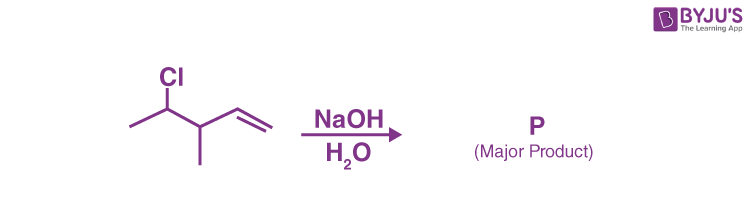Consider the above reaction. The number of π electrons present in the product ‘P’ is_________.

Sol.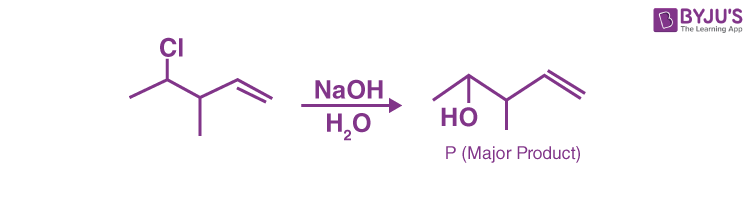The given reaction undergoes nucleophilic substitution by SN2 mechanism at room temperature

∴ No. of π electrons present in P = 2

10. In alanylglycylleucylalanyvaline, the number of peptide linkages is __________.,